# Editing Equations in Oxygen XML Editor

Editing equations in Oxygen XML Editor version 14.0 was more difficult than we expected. After trying several of the leading MathML editors, we found a versatile, easy-to-use, inexpensive solution.

Oxygen XML Fragment Editor

The Help Pages of Oxygen XML Editor version 14.0 have this to say about its support for equations:

“… includes a built-in editor for MathML notations. To start the MathML editor either double click a MathML notation or select the Edit Equation action from its contextual menu. In the MathML editor you are able to edit the mathematical symbols of a MathML notation.”

Either action opens the XML Fragment Editor window. A few problems with using this tool will surprise the user:

1. WYSIWYG tools for entering new equations are not available.

MathML is not intended to be written or edited directly; an important reason all XML editors include a wysiwyg editing mode for MathML. This mode in Oxygen is called Authoring and is selected using the Author tab. While in Authoring mode, an equation is inserted by selecting Dita->Insert-> Insert Equation from the contextual menu. This action opens the XML Fragment Editor window with the Pythagorean equation as its default. The MathML code that implements this equation is displayed directly below the equation (see the example below). Note that there are no controls or menus in the window for rewriting the equation.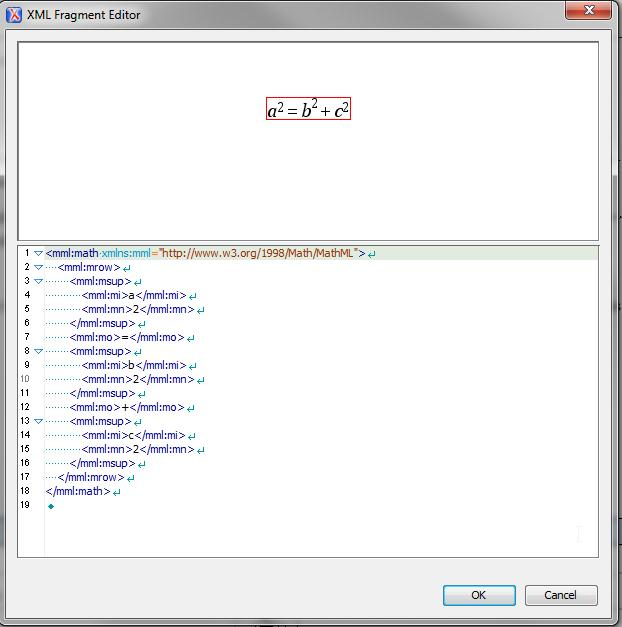2. It is difficult, if not impractical, to edit equations using its wysiwyg feature.

The red box enclosing the equation is the only hint of possible wysiwyg editing capability. You can point-and-click on a component of this equation so the red box surrounds it and then make a simple change in code below it. For example, you can select each “squared” term and change it to “cubed”. Or you can change the “+” to “-“. But you cannot insert complex operators.

3. Although the bottom half of the window allows editing an equation via its MathML code, the code quickly becomes intractable as expressions become more complex.

The MathML displayed in the XML Fragment Editor window is the same non-wysiwyg view as would be seen if you had selected the Text tab. The user could, in theory, enter a new equation by making changes to the default equation in Text mode. But, as has been said, MathML is not intended to be written or edited directly. The complexity of the code just for the default equation makes this apparent. Also, you can also see the difficulty of locating components to make even simple edits in an existing complex equation that someone else has entered into the document, as shown  in the screen capture below.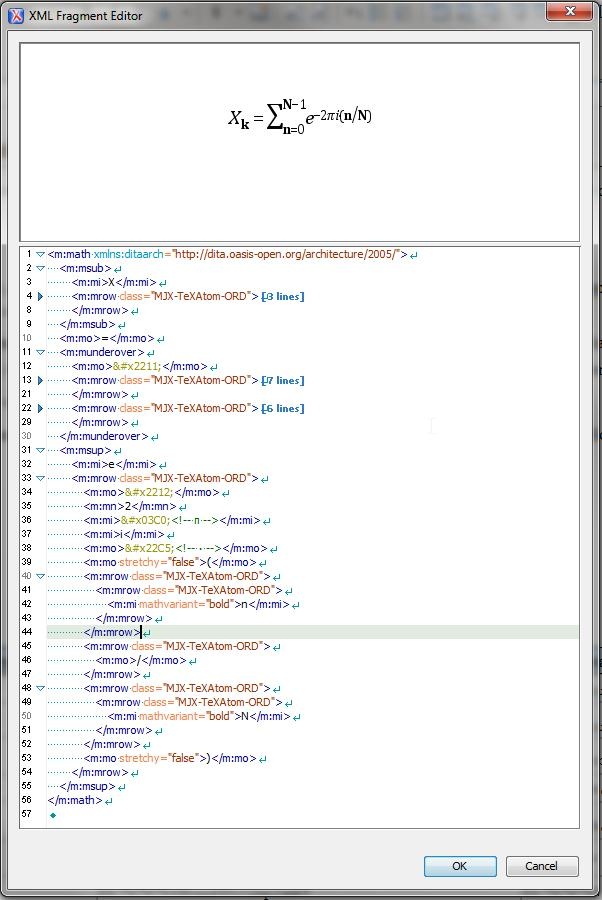Because we found the Oxygen XML Fragment Editor impractical for editing MathML, we researched other MathML editing options.

MathFlow

The makers of Oxygen XML Editor recommend the MathFlow plugin from Design Science for a fully functional MathML authoring tool.  The interface is easy to use and familiar to anyone who has entered equations in MSWord. However, the MathFlow plugin was incompatible with our client’s specialized build routine to output pdf and html failed, making the tool unusable for us.

Web Equation

At the Web Equation web-site (http://webdemo.visionobjects.com/equation.html?locale=default), an equation can be moused onto a grid, like drawing onto legal pad (see below). A print-quality image of the equation is rendered below the grid, to confirm the moused input. The MathML code is displayed by clicking on the MathML tab. This code can then be copied from the web-site and pasted directly into the XML document while in Text mode. Or it can be pasted into the document while in Authoring mode via the XML Fragment Editor window. However, with either method, our XML schema requires “m:” prefixes that are missing from the code supplied by Web Equation. For example, <mrow> and </mrow> tags should be <m:mrow> and </m:mrow>. To solve this problem as efficiently as possible, we copied the code into Notepad, used Notepad’s Find/Replace function to replace all instances of <m and </m with <m:m and </m:m, then copied-pasted this result into the XML document.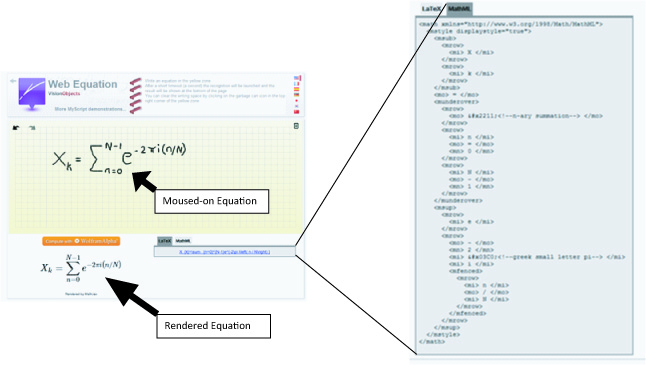We found Web Equation to be of limited use, not just because of the extra step needed to add element prefixes, but also because many attempts were needed before the rendered equation was correct.

Mathematics StackExchange

Mathematics StackExchange is a mathematics question and answer website (http://math.stackexchange.com/search?q=serial+summation). We searched and found summation equations similar to what we wanted. The MathML code for any equation at that website can be displayed by right-clicking the equation. Then the code is entered into the XML document using the same steps as described for Web Equation. Of course, because it is unlikely that any of the equations available at Mathematics StackExchange will be an exact match to what is needed, editing is generally required, which is a non-trivial effort using either Notepad or the Oxygen editor.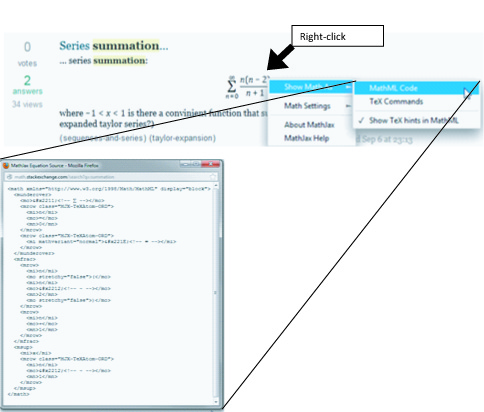MathType

MathType, also from Design Science, has the same user-friendly interface as MathFlow and its MathML coding includes compatible namespace prefixes. The user enters the equation using a complete set of mathematic operators and templates displayed at the top of the MathType window, then only has to copy the equation rendered in the window and paste directly into the XML document. Our build routine worked perfectly. We chose MathType  as our primary MathML editor because it provides all the functionality we need and, because it is not a plugin, doesn’t break the Oxygen editor.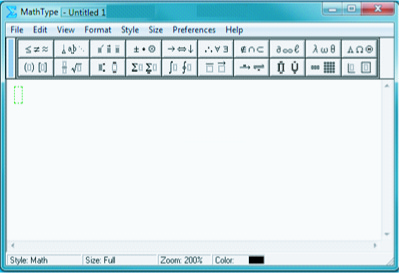Grandy is a senior technical writer at Phoenix Technical Publications. Phoenix Tech Pubs has provided complete technical writing and documentation services in Oakland and the San Francisco Bay Area for over 25 years.